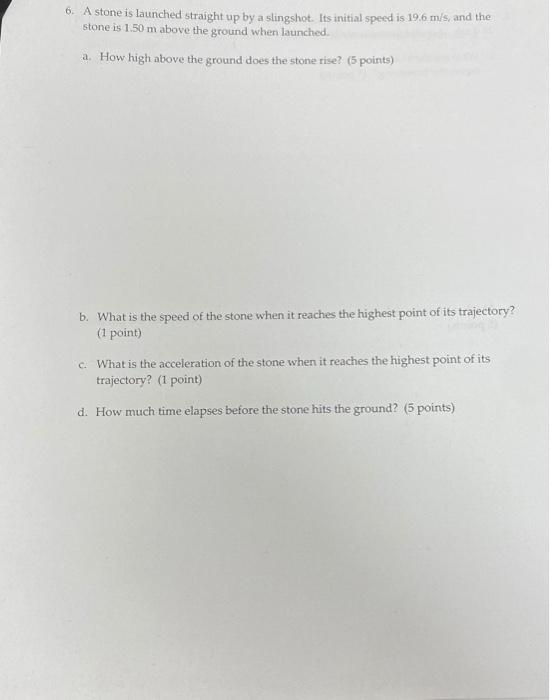# Question Solved1 Answer6. A stone is launched straight up by a slingshot. Its initial speed is 19.6 m/s, and the stone is 1.50 m above the ground when launched. a. How high above the ground does the stone rise? (5 points) b. What is the speed of the stone when it reaches the highest point of its trajectory? (1 point) What is the acceleration of the stone when it reaches the highest point of its trajectory? (1 point) d. How much time elapses before the stone hits the ground? (5 points)Transcribed Image Text: 6. A stone is launched straight up by a slingshot. Its initial speed is 19.6 m/s, and the stone is 1.50 m above the ground when launched. a. How high above the ground does the stone rise? (5 points) b. What is the speed of the stone when it reaches the highest point of its trajectory? (1 point) What is the acceleration of the stone when it reaches the highest point of its trajectory? (1 point) d. How much time elapses before the stone hits the ground? (5 points)
More
Transcribed Image Text: 6. A stone is launched straight up by a slingshot. Its initial speed is 19.6 m/s, and the stone is 1.50 m above the ground when launched. a. How high above the ground does the stone rise? (5 points) b. What is the speed of the stone when it reaches the highest point of its trajectory? (1 point) What is the acceleration of the stone when it reaches the highest point of its trajectory? (1 point) d. How much time elapses before the stone hits the ground? (5 points)